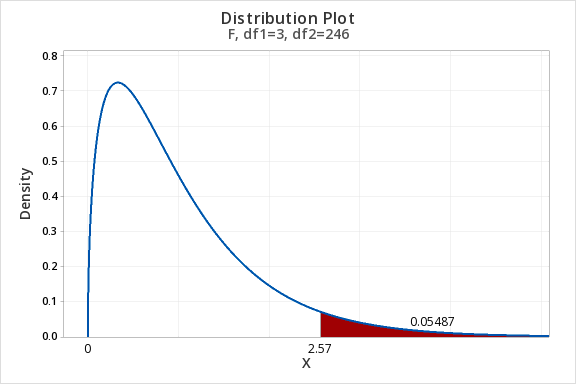# 10.1 - Introduction to the F Distribution

10.1 - Introduction to the F Distribution

One-way ANOVAs, along with a number of other statistical tests, use the F distribution. Earlier in this course you learned about the $$z$$ and $$t$$ distributions. You computed $$z$$ and $$t$$ test statistics and used those values to look up p-values using statistical software. Similarly, in this lesson you are going to compute F test statistics. The F test statistic can be used to determine the p-value for a one-way ANOVA.

The video below gives a brief introduction to the F distribution and walks you through two examples of using Minitab to find the p-values for given F test statistics. The steps for creating a distribution plot to find the area under the F distribution are the same as the steps for finding the area under the $$z$$ or $$t$$ distribution. For the F distribution we will always be looking for a right-tailed probability. Later in this lesson we will see that this area is the p-value.

The F distribution has two different degrees of freedom: between groups and within groups. Minitab will call these the numerator and denominator degrees of freedom, respectively. Within groups is also referred to as error.

Between Groups (Numerator) Degrees of Freedom

$$df_{between}=k-1$$

$$k$$ = number of groups

Within Groups (Denominator, Error) Degrees of Freedom

$$df_{within}=n-k$$

$$n$$ = total sample size with all groups combined

$$k$$ = number of groups

## Minitab® – Creating an F Distribution

Scenario: An F test statistic of 2.57 is computed with 3 and 246 degrees of freedom. What is the p-value for this test?

We can create a distribution plot. Our distribution is the F distribution. The numerator df ($$df_1$$) is 3 and the denominator df ($$df_2$$) is 246. We want to shade the area in the right tail. Our “X Value” is 2.57.

1. Open Minitab
2. Select Graph > Probability Distribution Plot > View Probability
3. Change the Distribution to F
4. Fill in the Numerator degrees of freedom with 3 and the Denominator degrees of freedom with 246
5. Select the Options button
6. Select A specified x value
7. Use the default Right tail
8. For the X value enter 2.57
9. OK and OKThe area beyond an F-value of 2.57 with 3 and 246 degrees of freedom is 0.05487. The p-value for this F test is 0.05487.

Note: When you conduct an ANOVA in Minitab, the software will compute this p-value for you.

  Link ↥ Has Tooltip/Popover Toggleable Visibility